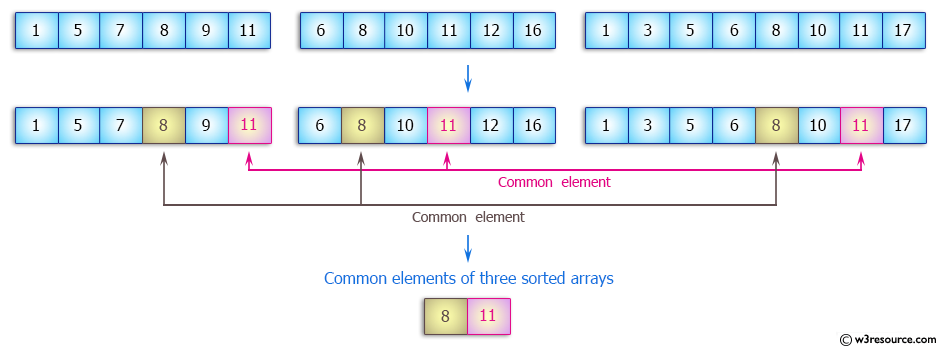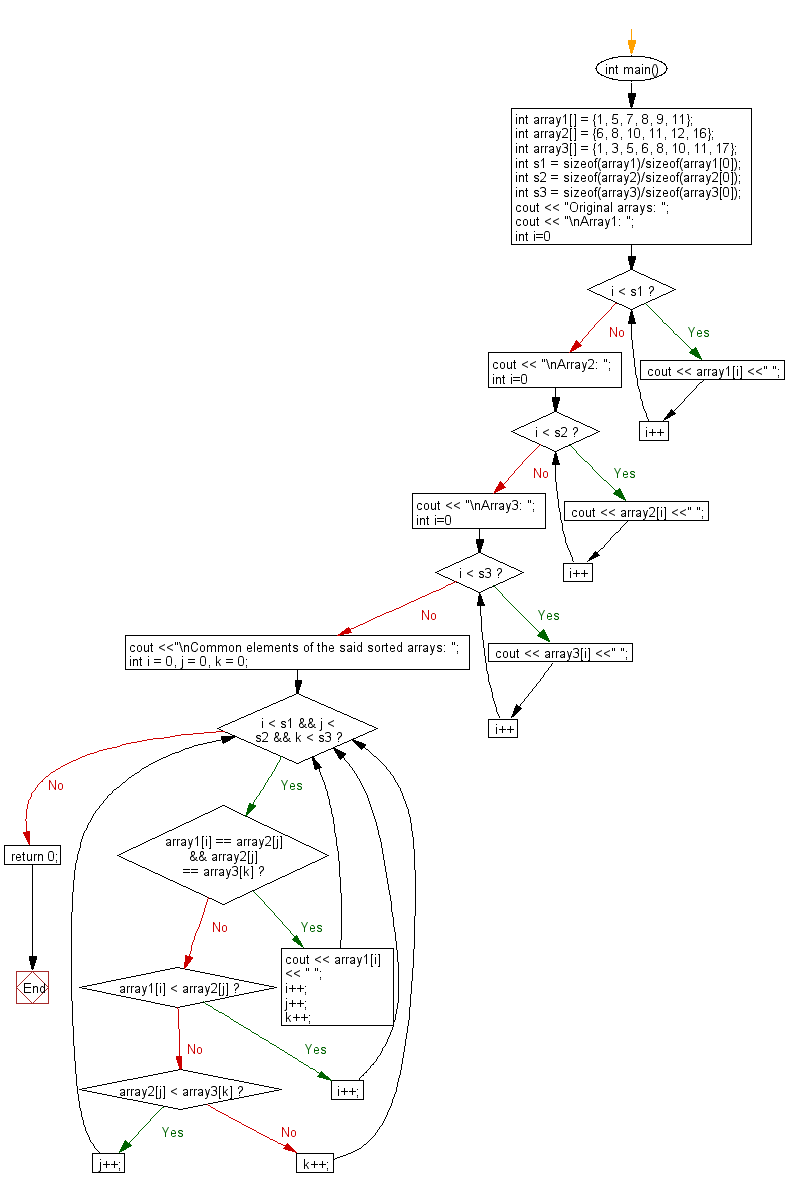﻿ C++ : Find all common elements in three sorted arrays# C++ Exercises: Find and print all common elements in three sorted arrays of integers

## C++ Array: Exercise-25 with Solution

Write a C++ program to find and print all common elements in three sorted arrays of integers.

Pictorial Presentation:Sample Solution:

C++ Code :

``````#include <iostream>
using namespace std;
int main()
{
int array1[] = {1, 5, 7, 8, 9, 11};
int array2[] = {6, 8, 10, 11, 12, 16};
int array3[] = {1, 3, 5, 6, 8, 10, 11, 17};

int s1 = sizeof(array1)/sizeof(array1);
int s2 = sizeof(array2)/sizeof(array2);
int s3 = sizeof(array3)/sizeof(array3);

cout << "Original arrays: ";
cout << "\nArray1: ";
for (int i=0; i < s1; i++)
cout << array1[i] <<" ";
cout << "\nArray2: ";
for (int i=0; i < s2; i++)
cout << array2[i] <<" ";
cout << "\nArray3: ";
for (int i=0; i < s3; i++)
cout << array3[i] <<" ";
cout <<"\nCommon elements of the said sorted arrays: ";
int i = 0, j = 0, k = 0;
while (i < s1 && j < s2 && k < s3)
{
if (array1[i] == array2[j] && array2[j] == array3[k])
{
cout << array1[i] << " ";
i++;
j++;
k++;
}

else if (array1[i] < array2[j])
i++;

else if (array2[j] < array3[k])
j++;

else
k++;
}
return 0;
}
``````

Sample Output:

```Original arrays:
Array1: 1 5 7 8 9 11
Array2: 6 8 10 11 12 16
Array3: 1 3 5 6 8 10 11 17
Common elements of the said sorted arrays: 8 11
```

Flowchart:C++ Code Editor:

What is the difficulty level of this exercise?

Test your Programming skills with w3resource's quiz.

﻿